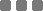## Wednesday, June 29, 2016

### Math: How many perfect squares are divisors of the product 1! • 2! • 3! • 4! • 5! • 6! • 7! • 8! • 9!?

This is from 2003 AMC (American Mathematics Competitions).

The problem looks like this:

How many perfect squares are divisors of the product 1! • 2! • 3! • 4! • 5! • 6! • 7! • 8! • 9!?
(A) 504 (B) 672 (C) 864 (D) 936 (E) 1008

The exclamation after the number means factorial. For instance, 3! = 1 • 2 • 3 = 6.

Let's break the sequence

The multiplication of that factorial sequence above looks like so:

Seriously, it looks like that.
I use middle dot (•) as the multiplication operator (usually ×) to make it consistent with the title above.

Wut is perfect square?

It's a number (prime or composite -- in this case, a positive integer) with (positive) even exponent (0 is also "even").
For example: 22 or 64 or 140 and such.

Let's "prime" it

The sequence above can be grouped into only prime numbers (such as in prime factorization).
As you can see, the prime parts are 2, 3, 5, and 7. The others (besides 1) are composites, let them be. We're gonna take just the primes.

We wanna construct them into:

2 a • 3 b • 5 c • 7 d

This will be useful because later we can find the ones with even exponent (perfect square) much easier.

The a (exponent for 2)

Take a look the sequence above vertically, and find all numbers which have "2" in them.
For purely number "2", we have 8.
For number "4", we have 6. Since 4 = 2 • 2, then we have another 12 (6 × 2) of "2".
For number "6", we have 4. Since 6 = 2 • 3, then we have another 4 of "2".
For number "8", we have 2. Since 8 = 2 • 2 • 2, then we have another 6 (2 × 3) of "2".
So then, all of probable factors (or the maximum exponent) of "2" is 8 + 12 + 4 + 6 = 30.

Thus, the a is 30.

The b (exponent for 3)

Let's find all numbers which have "3".
For purely number "3", we have 7.
For number "6", we have 4. Since 6 = 2 • 3, then we have another 4 of "3".
For number "9", we have 2. Since 9 = 3 • 3, then we have another 4 (2 × 2) of "3".
So then, total exponent for "3" is 7 + 4 + 2 = 13.

Thus, the b is 13.

The c (exponent for 5)

Let's find all numbers which have "5".
For purely number "5", we have 5.
That's it, nothing else.

Thus, the c is 5.

The d (exponent for 7)

Let's find all numbers which have "7".
For purely number "7", we have 3.
And that's it.

Thus, the d is 3.

Therefore,

1! • 2! • 3! • 4! • 5! • 6! • 7! • 8! • 9! can also be written as:

2 30 • 3 13 • 5 5 • 7 3

The perfect square part from each

We are only interested in the even ones.

• For "2", the exponent is 30, it has 16 even parts: 0, 2, 4, 6, 8, 10, 12, ..., 30.
• For "3", the exponent is 13, it has 7 even parts: 0, 2, 4, 6, 8, 10, 12.
• For "5", the exponent is 5, it has 3 even parts: 0, 2, 4.
• For "7", the exponent is 3, it has 2 even parts: 0, 2.

Multiply all the even ones

The answer is 16 • 7 • 3 • 2 = 672 (the B option).

It means there are 672 perfect squares that can be used to divide the thingy above without resulting remainder.

Why multiply? Because it is a multiplication combinatoric pattern, so we multiply them. Hm.
Like for instance, the multiplication of 24 and 52 can be used to divide the product of the dilly.

This problem is about observing pattern and then doing the algebraic maneuver that fits the pattern.

This can be proven with smaller sequence.
For example:

• 1! • 2! • 3! ► total of perfect square divisors for the multiplication product is 2 (1 and 4).
• 1! • 2! • 3! • 4! ► total of perfect square divisors for the multiplication product is 6 (1, 4, 9, 16, 36, and 144 -- we cannot form "25"/"49"/"64"/others from the sequence).
• Et al...Math: How many perfect squares are divisors of the product 1! • 2! • 3! • 4! • 5! • 6! • 7! • 8! • 9!?
https://monkeyraptor.johanpaul.net/2016/06/math-how-many-perfect-squares-are_29.html